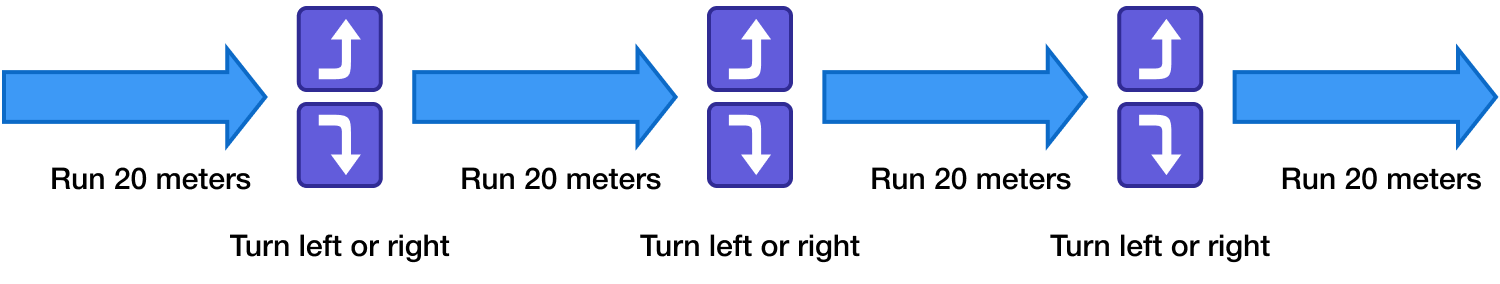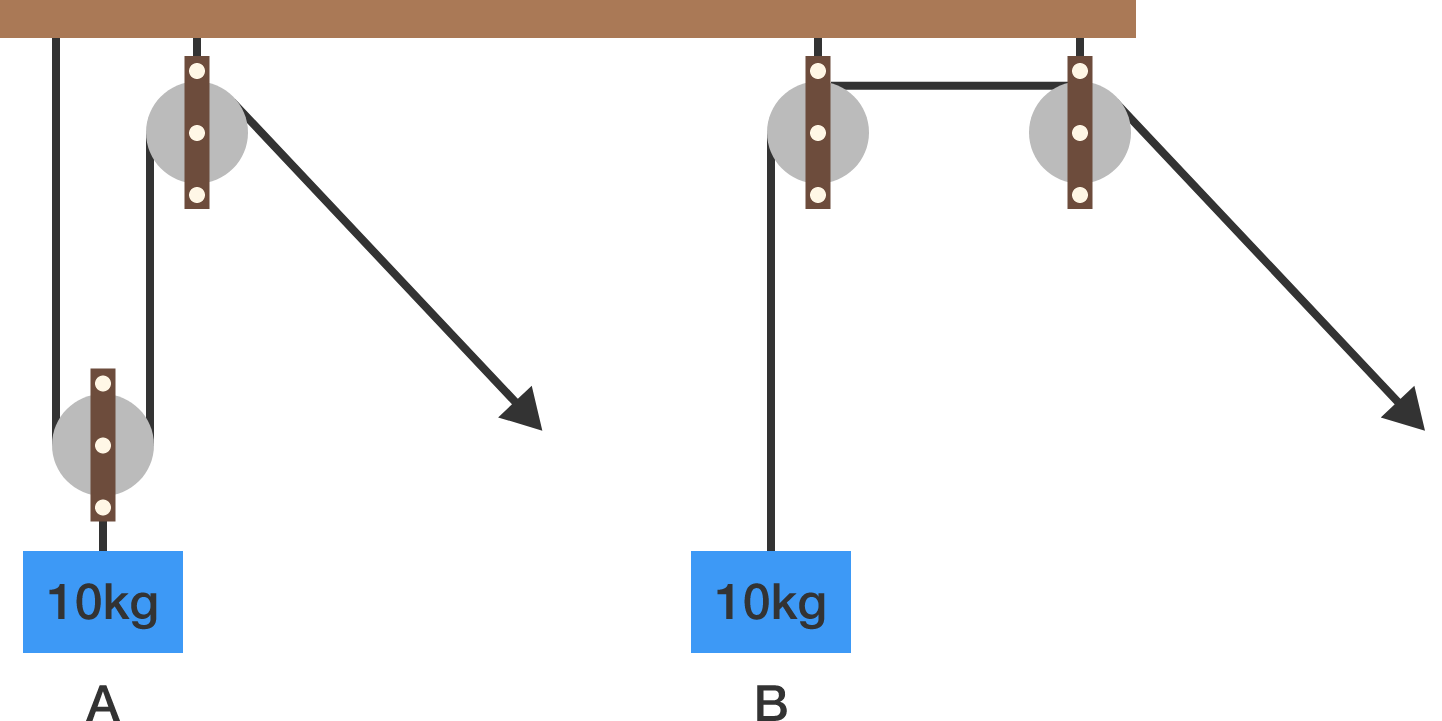# 2017-02-20 IntermediateDodgy Daryl is running away from a horde of zombies. To escape, he runs 20 meters and then turns $90^\circ$ to the left or to the right.

Just before the fourth turn, what is his maximum possible displacement from the start?

In which arrangement of pulleys given below do we need less force to lift the weight?Details and Assumptions:

• The pulleys are massless.
• The friction is absent everywhere.

$\large \lim_{x\to 0^+} x = 0 \qquad \qquad \lim_{x\to0^+} x^x = 1$

The two limits above are commonly used in calculus textbooks. However, the following limit isn't! What is the value of this limit?

$\large \lim_{x\to0^+} x^{x^x}$

$\big(n^2 + 2n + 2\big) \, \Big| \, \big(n^3 + 4n^2 + 4n - 14\big)$ Find the sum of all integers $n$ such that the above holds true.


Notation: $a \mid b$ means $a$ is a factor of $b$.

In the game of M2S3, the goal is to use minimum number of steps to get from one number to the next. In each step, you can either

• multiply by 2, or
• subtract 3.

For example, if we wanted to get from 8 to 11, we could do so in 5 steps:

$\large 8 \overset{-3}{\longrightarrow} 5 \overset{\times2}{\longrightarrow}10 \overset{-3}{\longrightarrow} 7\overset{\times2}{\longrightarrow}14 \overset{-3}{\longrightarrow}11.$

What is the minimum possible number of steps required to get from 11 to 25?

×

Problem Loading...

Note Loading...

Set Loading...